# WARMUP GRAPHING INEQUALITIES Inequality symbols less than fewer

• Slides: 9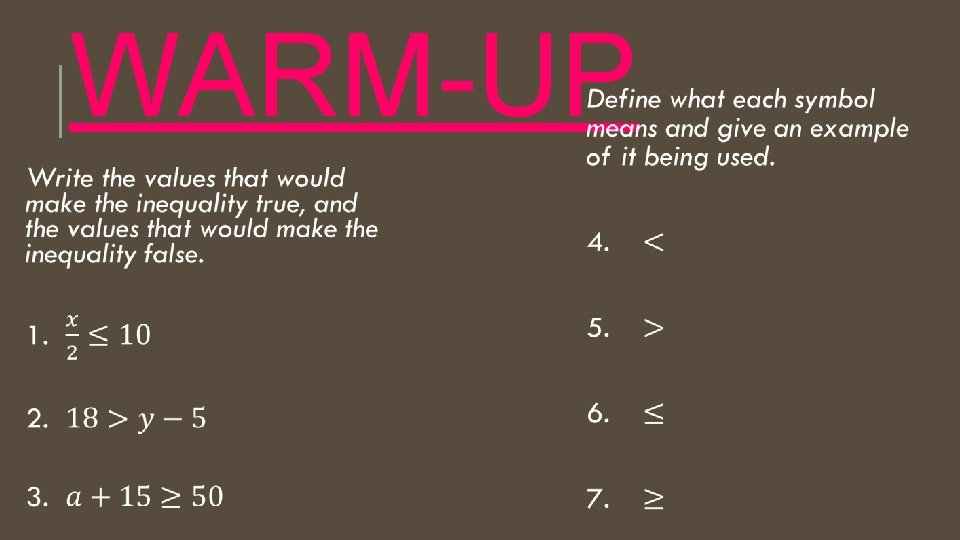WARM-UPGRAPHING INEQUALITIES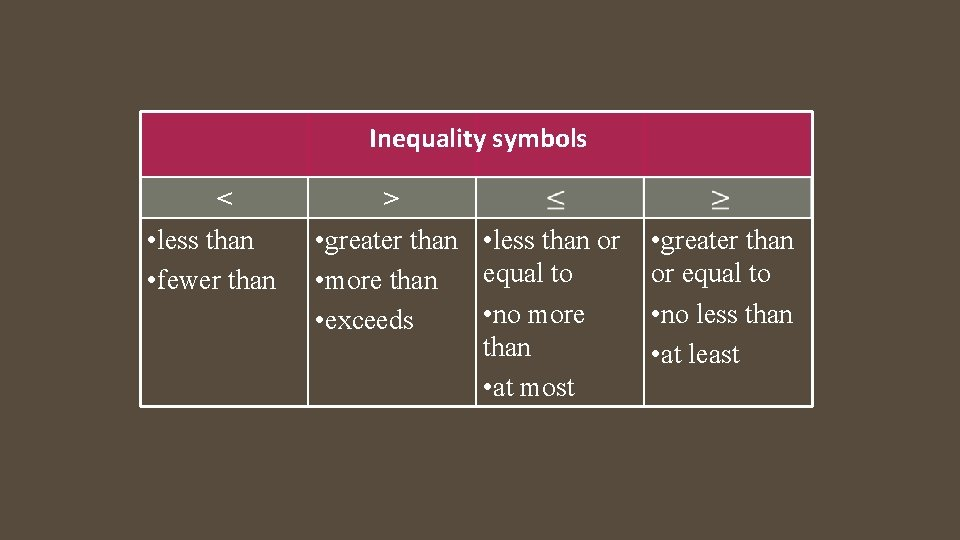Inequality symbols < • less than • fewer than > • greater than • less than or equal to • more than • no more • exceeds than • at most • greater than or equal to • no less than • at least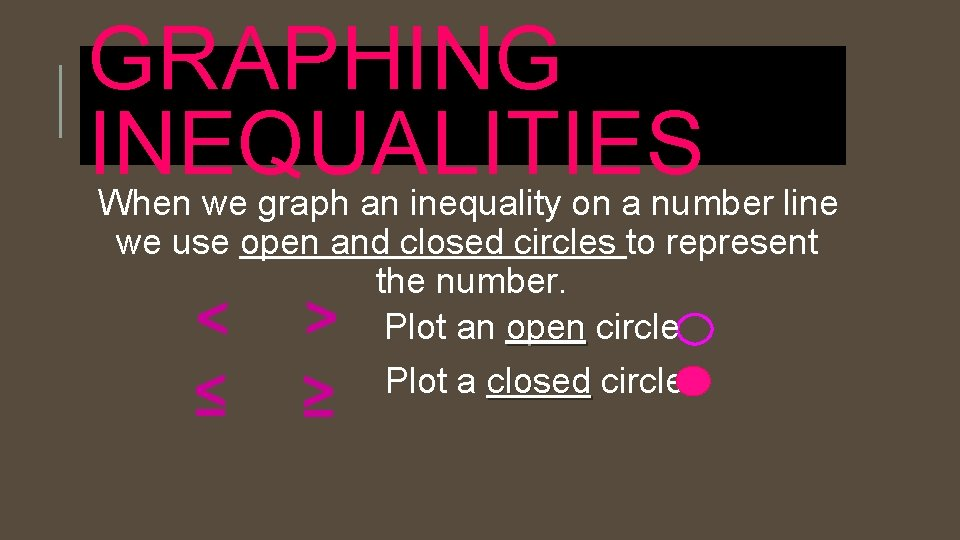GRAPHING INEQUALITIES When we graph an inequality on a number line we use open and closed circles to represent the number. Plot an open circle Plot a closed circle < < ≤ ≥Graphing Inequalities x < c When x is less than a constant, you darken in the part of the number line that is to the left of the constant. Also, because there is no equal line, we are not including where x is equal to the constant. That means we are not including the endpoint. One way to notate that is to use an open hole at that point. x > c When x is greater than a constant, you darken in the part of the number line that is to the right of the constant. Also, because there is no equal line, we are not including where x is equal to the constant. That means we are not including the endpoint. One way to notate that is to use an open hole at thatx < c When x is less than or equal to a constant, you darken in the part of the number line that is to the left of the constant. Also, because there is an equal line, we are including where x is equal to the constant. That means we are including the endpoint. One way to notate that is to use an closed hole at that point. x > c When x is greater than or equal to a constant, you darken in the part of the number line that is to the right of the constant. Also, because there is an equal line, we are including where x is equal to the constant. That means we are including the endpoint. One way to notate that is to use a closed hole at that point.Graphing Inequalities. Graph each of these inequalities. 8. State the inequality represented on the number line below.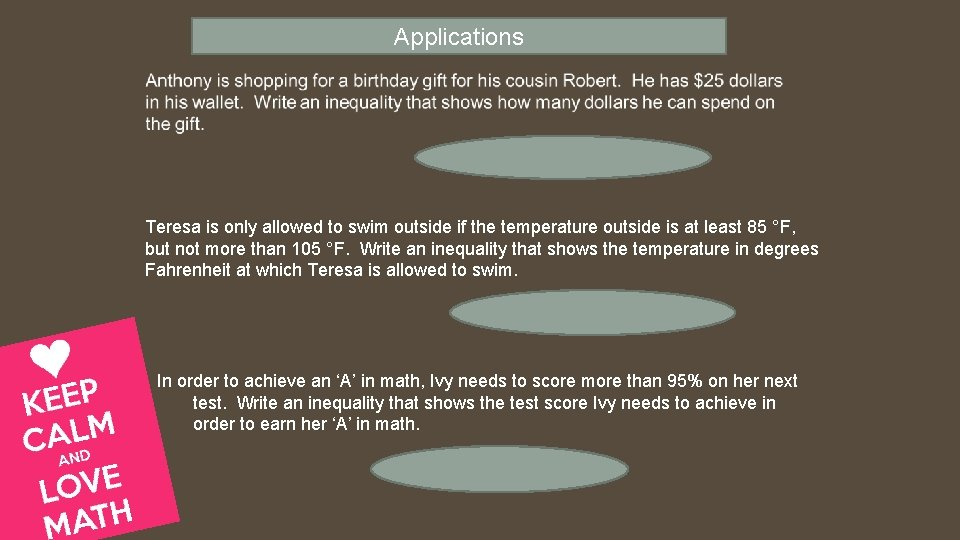Applications Teresa is only allowed to swim outside if the temperature outside is at least 85 °F, but not more than 105 °F. Write an inequality that shows the temperature in degrees Fahrenheit at which Teresa is allowed to swim. In order to achieve an ‘A’ in math, Ivy needs to score more than 95% on her next test. Write an inequality that shows the test score Ivy needs to achieve in order to earn her ‘A’ in math.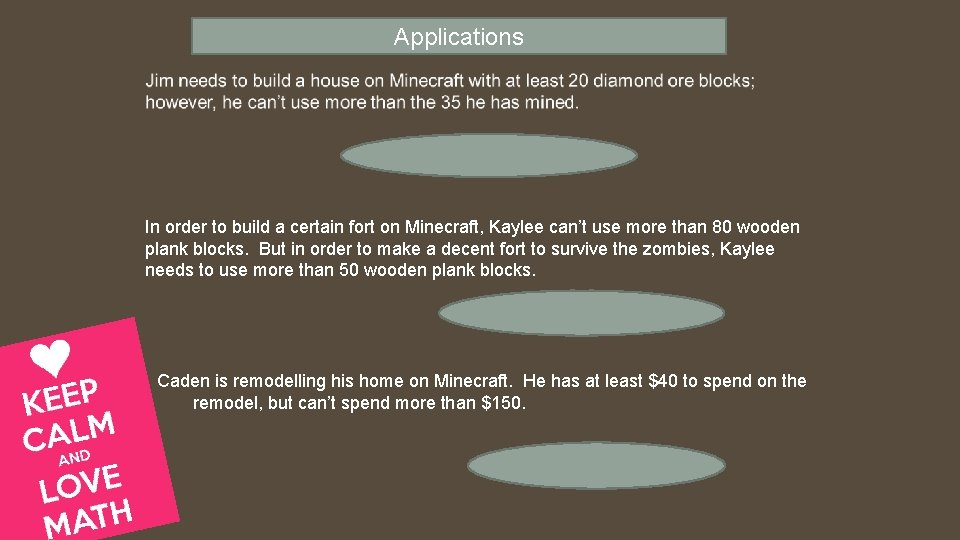Applications In order to build a certain fort on Minecraft, Kaylee can’t use more than 80 wooden plank blocks. But in order to make a decent fort to survive the zombies, Kaylee needs to use more than 50 wooden plank blocks. Caden is remodelling his home on Minecraft. He has at least \$40 to spend on the remodel, but can’t spend more than \$150.